# Sigma Notation

Sigma notation is used to hold all the terms of a series on one small space on a page.

Take for example the sequence. This sequence has general term. The notation: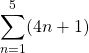is the instruction to add together the first five terms of the sequence. It reads ‘sum the terms of the sequencestarting atand ending at.’

Writing out the sum in full we have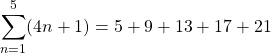We can also understand this notation as representing the number or expression which the sum is equal to. In this case,. Therefore,# Evaluate Sigma Notation

There are three ways to calculate the value of (evaluate) a sum represented with sigma notation.

1. Write out all the terms and add them together.
2. If you know a formula for the kind of sequence, use the formula.
3. Use a scientific calculator, or an online sigma calculator.

# Using formulae

## Example 1 Arithmetic SeriesIn this case, the sequenceis arithmetic, and so we can calculate the sum of the first 100 terms using the formula for arithmetic series:whereis the first term andis the common difference.

The sequencehas first termand common difference.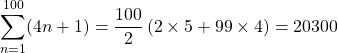It is clear that having a formula offers a significant advantage over writing out all the terms and adding them together.

Try typing example 1 into this sigma calculator.

## Example 2: A partial sumIn this example, our sequence is still arithmetic, however we are beginning at the 7th term and finishing at the 20th term. That is a total of 14 terms. (Calculate:We ‘‘ because the 7th term is included).

Now that we have established, let’s find the first term in this summation and the last term, so that we can use the other formula for summing an arithmetic sequence which isFirst term: when,.

Last term: when,.

Therefore,## Example 3 A geometric series

A geometric sequence has general term. We read here that the formula to sum the firstterms of a geometric sequence is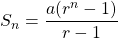In this case, the number of terms is 10;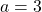and.

When written out, the sequence readsUsing the formula, we have## Example 4 Square Numbers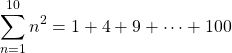In this course, we don’t derive the formula to add together the square numbers. It takes a little more time to derive this formula – here is one youtube that explains where the formula comes from.

Here it is:Therefore,## Example 5 Summingterms

When the number of terms to add has not been specified, we generally useto describe the number of terms to be added. This means that we need an additional variable to use to describe the sequence. Let’s add togetherterms of the sequence in example 1:This reads ‘sum the terms of the sequencestarting with the first term and ending with theterm.

Writing the sequence out we have:This sequence is arithmetic, withterms,and.Before evaluating a sum presented with sigma notation, it is good to ask whether or not a formula would be necessary. If only a few terms are to be added, it might be more efficient to write out the terms and add them. If there are many terms and you are not permitted to use technology, then you then need to determine the kind of sequence in order to select the correct formula for adding the terms.

# Simplifying Sigma Notation

Consider the sum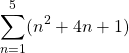Written out in full we haveRearranging the terms, taking all the first terms in each bracket followed by all the second terms, followed by all the third we have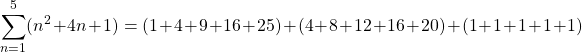We can identify three separate, simpler sums:Taking out the common factorin the second term, we can simplify once more:Finally, notice that the constant term is simply a multiplication:We can make the following generalizations:

1. Letandrepresent two sequences in terms of. Then:2. Letbe a sequencemultiplied by a constant.3. Letbe a constant.# Practice

Next: Sum to Infinity